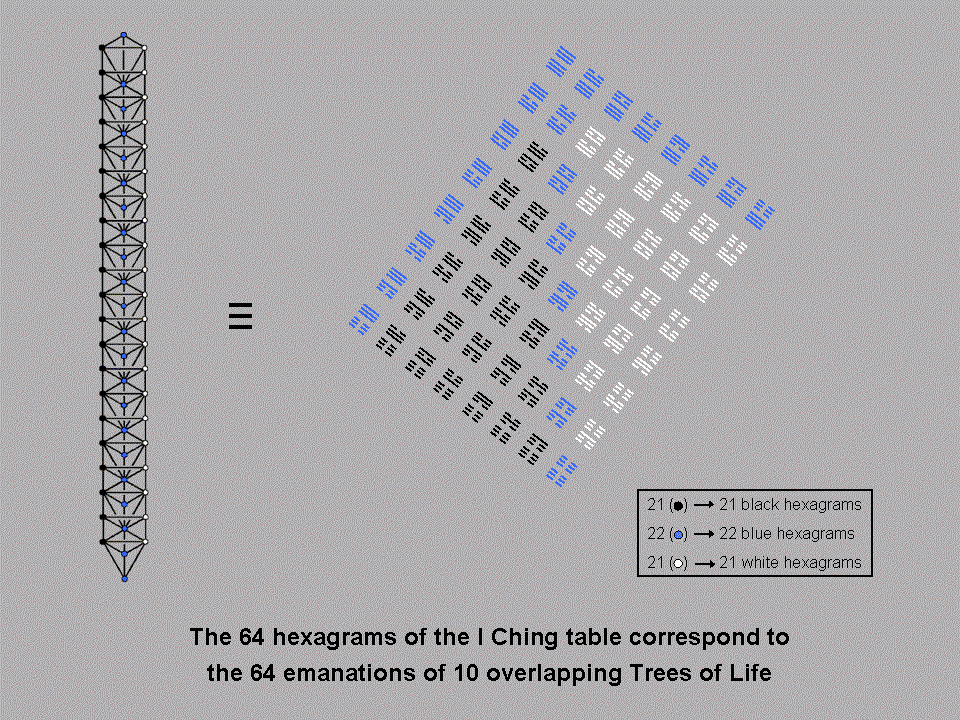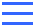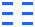Home

 << Previous    1...   11  12    14  15  ...39    Next >>#13 Correspondence between the 10-tree and the 64 hexagrams

Replacing each of the ten Sephiroth in the Tree of Life by another Tree of Life generates ten overlapping Trees of Life with 64 'Sephirothic levels' (SLs). 64 is the number value of Nogah, the Mundane Chakra of the Sephirah Hod, signifying mental activity and communication (see Table 1). 21 SLs (denoted by black dots) line the left-hand Pillar of Judgement, 21 SLs (denoted by white dots) line the right-hand Pillar of Mercy and 22 SLs (denoted by blue dots) line the central Pillar of Equilibrium. 21 is the number value of EHYEH (אהיה), the Godname of Kether.

Compare this with the 8×8 array of hexagrams that form the basis of the ancient Chinese I Ching system of divination. It consists of 64 hexagrams. 22 blue ones line two adjacent sides and a diagonal, 21 black hexagrams form a right-angled triangle on one side of the diagonal and 21 white hexagrams form a right-angled triangle on its opposite side. The hexagram (Chien) in the top left-hand corner of the table is a pair of "Heaven" trigrams:. This hexagram corresponds to the first SL, namely, Kether of the tenth Tree of Life. The hexagram (K'un) in the bottom right-hand corner is a pair of "Earth" trigrams:. It corresponds to the last SL, namely, Malkuth of the first Tree of Life, whose Mundane Chakra is the planet Earth. The 15 blue hexagrams on adjacent sides of the I Ching table correspond to the uppermost 15 SLs on the Pillar of Equilibrium. The remaining seven blue hexagrams on the diagonal correspond to the lowest seven SLs on this pillar that belong to the two lowest Trees. This 15:7 division of hexagrams reflects a 8:2 division of 10 overlapping Trees.

Superstrings
In terms of superstring theory, the 10 Trees represent dimensions of the 10-dimensional space-time that this theory predicts, the lowest Tree denoting the dimension of time. Therefore, the two lowest Trees of Life denote time and a large-scale dimension of space — the so-called "longitudinal" dimension, along which distance along the length of the superstring is measured. The eight highest Trees of Life represent the eight so-called "transverse" dimensions — the dimensions of space perpendicular to the superstring at any point along its length. So the 15:7 division referred to above reflects the primary division of superstring space-time into the eight transverse dimensions, the longitudinal dimension and time. The same pattern manifests in the 10 superstring dimensions and the I Ching table of hexagrams because the former constitute a holistic system represented by the latter and —par excellence — the tetractys (see here). YAH (יה), the (older) Godname of Chokmah, prescribes this division because its number value is 15.

 << Previous    1...   11  12    14  15  ...39    Next >>

Home